# DAV Class 8 Maths Chapter 12 Worksheet 4 Solutions

The DAV Maths Class 8 Solutions and DAV Class 8 Maths Chapter 12 Worksheet 4 Solutions of Construction of Quadrilaterals offer comprehensive answers to textbook questions.

## DAV Class 8 Maths Ch 12 WS 4 Solutions

Question 1.
Using a ruler and compasses only, construct the following:
(i) A quadrilateral ABCD in which $$\overline{\mathrm{AB}}$$ = $$\overline{\mathrm{CD}}$$ = 5 cm, $$\overline{\mathrm{BC}}$$ = 4.5 cm, ∠B = 45°, ∠C = 135°.
Solution:
Steps of Construction:
1. Draw $$\overline{\mathrm{AB}}$$ = 5 cm.
2. Draw an angle of 45° at B and cut $$\overline{\mathrm{BC}}$$ = 4.5 cm.
3. Draw an angle of 135° at C and cut $$\overline{\mathrm{CD}}$$ = 5 cm.
4. Join $$\overline{\mathrm{AD}}$$.
5. ABCD is the required quadrilateral.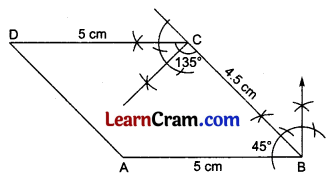(ii) A quadrilateral ABCD in which $$\overline{\mathrm{AB}}$$ = 5 cm, $$\overline{\mathrm{BC}}$$ = 6 cm, $$\overline{\mathrm{CD}}$$ = 6.5 cm, ∠B = 105°, ∠C = 75°.
Solution:
Steps of Construction:
1. Draw $$\overline{\mathrm{AB}}$$ = 5 cm.
2. Draw an angle of 105° at B and cut $$\overline{\mathrm{BC}}$$ = 6 cm.
3. Draw an angle of 75° at C and cut $$\overline{\mathrm{CD}}$$ = 6.5 cm.
4. Join D to A.
5. ABCD is the required quadrilateral.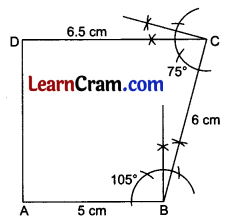(iii) A quadrilateral PQRS in which $$\overline{\mathrm{PQ}}$$ = 5 cm, $$\overline{\mathrm{QR}}$$ = 4 cm, $$\overline{\mathrm{RS}}$$ = 4.5 cm, ∠Q = 90°, ∠R = 135°.
Solution:
Steps of Construction:
1. Draw $$\overline{\mathrm{PQ}}$$ = 5 cm.
2. Draw an angle of 90° at Q and cut $$\overline{\mathrm{QR}}$$ = 4.5 cm.
3. Draw an angle of 135° at R and cut $$\overline{\mathrm{RS}}$$ = 4.5 cm.
4. Join S to P.
5. PQRS is the required quadrilateral.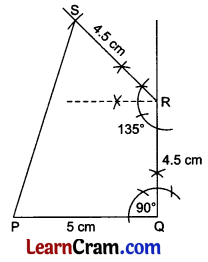(iv) A quadrilateral MNRS in which $$\overline{\mathrm{MN}}$$ = 4.5 cm, $$\overline{\mathrm{NR}}$$ = 5 cm, $$\overline{\mathrm{RS}}$$ = 6 cm, ∠N = 120°, ∠R = 60°.
Solution:
Steps of Construction:
1. Draw $$\overline{\mathrm{MN}}$$ = 4.5 cm.
2. Draw an angle of 120° at N and cut $$\overline{\mathrm{NR}}$$ = 5 cm.
3. Draw an angle of 60° at R and cut $$\overline{\mathrm{RS}}$$ = 6 cm.
4. Join S to M.
6. MNRS is the required quadrilateral.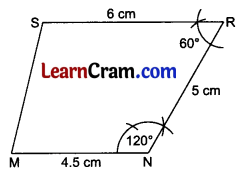(v) A quadrilateral ABCD in which $$\overline{\mathrm{AB}}$$ = $$\overline{\mathrm{BC}}$$ = $$\overline{\mathrm{CD}}$$ = 6 cm and ∠B = 60°, ∠C = 120°.
Solution:
1. Draw $$\overline{\mathrm{AB}}$$ = 6 cm.
2. Draw an angle of 60° at B and cut $$\overline{\mathrm{BC}}$$ = 6 cm.
3. Draw an angle of 120° at C and cut $$\overline{\mathrm{CD}}$$ = 6 cm.
4. Join D to A.
5. ABCD is the required quadrilateral.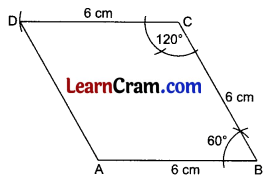Question 2.
Using a ruler, compasses, and protractor if required, construct the following:
(i) A quadrilateral ABCD in which $$\overline{\mathrm{AB}}$$ = 5 cm, $$\overline{\mathrm{BC}}$$ = 4 cm, $$\overline{\mathrm{CD}}$$ = 4.5 cm, ∠B = 70°, ∠C = 100°.
Solution:
Steps of Construction:
1. Draw $$\overline{\mathrm{AB}}$$ = 5 cm.
2. Draw an angle of 70° at B and cut $$\overline{\mathrm{BC}}$$ = 4 cm.
3. Draw an angle of 100° at C and cut $$\overline{\mathrm{CD}}$$ = 4.5 cm.
4. Join D to A.
5. ABCD is the required quadrilateral.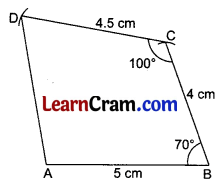(ii) A quadrilateral PQRS in which $$\overline{\mathrm{PQ}}$$ = 5 cm, $$\overline{\mathrm{QR}}$$ = 6 cm, $$\overline{\mathrm{RS}}$$ = 4 cm, ∠Q = 80°, ∠R = 100°.
Solution:
Steps of Construction:
1. Draw $$\overline{\mathrm{PQ}}$$ = 5 cm.
2. Draw an angle of 80° at Q and cut $$\overline{\mathrm{QR}}$$ = 6 cm.
3. Draw ∠R = 100° and cut $$\overline{\mathrm{RS}}$$ = 4 cm.
4. Join S to P.
5. PQRS is the required quadrilateral.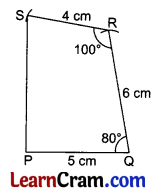(iii) A quadrilateral ABCD in which $$\overline{\mathrm{AB}}$$ = 4 cm, $$\overline{\mathrm{BC}}$$ = 5 cm, $$\overline{\mathrm{CD}}$$ = 4.5 cm, ∠B = 85°, ∠C = 95°.
Solution:
Steps of Construction:
1. Draw $$\overline{\mathrm{AB}}$$ = 4 cm.
2. Draw an angle of 85° with protractor at B and cut $$\overline{\mathrm{BC}}$$ = 5 cm.
3. Draw ∠C = 95° with protractor and cut $$\overline{\mathrm{CD}}$$ = 4.5 cm.
4. Join D and A.
5. ABCD is the required quadrilateral.(iv) A quadrilateral ABCD in which $$\overline{\mathrm{AB}}$$ = 4 cm, $$\overline{\mathrm{BC}}$$ = 3 cm, $$\overline{\mathrm{CD}}$$ = 4.5 cm, ∠B = 105°, ∠C = 80°.
Solution:
Steps of Construction:
1. Draw $$\overline{\mathrm{AB}}$$ = 4 cm.
2. Draw ZB = 105° and cut $$\overline{\mathrm{BC}}$$ = 3 cm.
3. Draw ZC = 80° and cut $$\overline{\mathrm{CD}}$$ = 4.5 cm.
4. Join D to A.
5. ABCD is the required quadrilateral.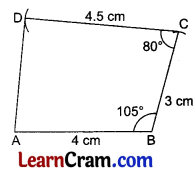(v) A quadrilateral ABCD in which $$\overline{\mathrm{AB}}$$ = 5 cm, $$\overline{\mathrm{BC}}$$ = 4 cm, $$\overline{\mathrm{CD}}$$ = 5 cm, ∠B = 80°, ∠C = 100°.
Solution:
Steps of Construction:
1. Draw $$\overline{\mathrm{AB}}$$ = 5 cm.
2. Draw ∠B = 80° and cut $$\overline{\mathrm{BC}}$$ = 4 cm.
3. Draw ∠C = 100° and cut $$\overline{\mathrm{CD}}$$ = 5 cm.
4. Join D and A.
5. ABCD is the required quadrilateral.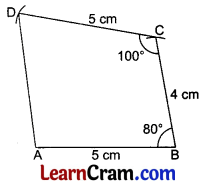### DAV Class 8 Maths Chapter 12 Value Based Questions

Question 1.
On a pastel sheet, construct a parallelogram of sides 45 cm and 30 cm and one of the base angles is 80°. Cut it out.
(i) Make a poster on ‘DIGITAL INDIA’, ‘MAKE IN INDIA’.
(ii) How can you contribute to ‘MAKE IN INDIA’.
Solution:
The parallelogram with given dimensions can be constructed with the following concepts.
We know that opposite sides and opposite angles of a parallelogram are equal and interior angles of the same side of a transversal are supplementary.
∴ The sides of the parallelogram will be 45 cm, 30 cm, 45 cm, and 30 cm.
One of the base angles = 80° (given)
Other base angle = 180° – 80° = 100°
∴ Angles of the Parallelogram will be 80°, 100°, 80°, 100°.
Now, the students can construct the required parallelogram on a pastel sheet themselves.
(i) Do yourself.
(ii) Making elders aware of the ‘Make in India’ concept and by identifying products that can be manufactured with a small budget we can contribute to ‘MAKE IN INDIA’.